# Introduction to the PyRETIS library¶

In this introduction to the PyRETIS library the main classes and functions from the PyRETIS library will be discussed. For the full description, we refer to the API documentation.

The PyRETIS library contains methods and classes that handle the different aspects of a simulation. These are grouped into sub-packages and the different sub-packages and the classes and methods defined within them will interact. As an example, assume that we are performing a RETIS simulation. In terms of objects from the PyRETIS library, this simulation can be described as follows:

1. We first define the System we are studying. This contains information about the Particles, Box and the ForceField.
2. Next, an OrderParameter is defined and the order parameter it is representing can be calculated using a System object as the argument.
3. The RETIS simulation is handled by the SimulationRETIS class which will use a specific EngineBase like object in order to generate several Path objects for a collection of PathEnsemble objects. This generation is done by a set of methods defined in the modules pyretis.core.tis and pyretis.core.retis.
4. An analysis is carried out by making use of methods from the analysis sub-package.

## The core and forcefield sub-packages¶

The pyretis.core library defines the core method and classes and here we will introduce the

Below is an illustration of how some of these classes are interacting. The classes (shown as boxes in this figure) will be discussed more in the following.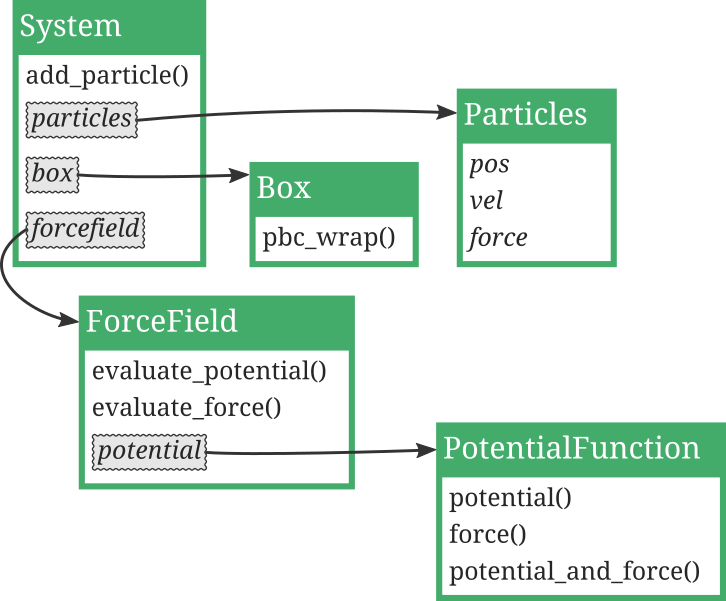Fig. 10 Illustration of the relations between classes from the core and force field sub-packages. The classes as shown as green boxes where the class names are shown with white text. Examples of class methods are written as “method()” while and class attributes are indicated as just “attribute”. Some of the attributes are in fact references to other classes and these are highlighted with grey boxes and the reference is shown by arrows. As can be seen in this figure, the System is in practice composed of several other classes.

### The System class¶

The System class defines the system we are investigating. It will typically contain particles, a simulation box and a force field. This class exposes important parts we can interact with, in particular, the particles.

Example of creation:

from pyretis.core import System
new_system = System(temperature=0.8, units='lj')


This will create an empty system with a set temperature equal to 0.8 in lj units (lj refers to Lennard-Jones units). It is also possible to specify a box here in case that it needed:

new_system = System(temperature=0.8, units='lj', box=mybox)


where mybox can be created as described below.

Particles can be added by first creating an object as described below. A short example:

from pyretis.core import System, Particles
new_system = System(temperature=0.8, units='lj')
new_system.particles = Particles()
new_system.add_particle([0.0, 0.0, 0.0], mass=1.0, name='Ar', ptype=0)


Here, we are setting the System.particles attribute and using the System.add_particle() to add a particle with a given position, mass, name and type.

### The Particles class¶

The Particles class represents a collection of particles and in many ways it can be viewed as a particle list. Internally in PyRETIS, the particle list is one of the most important classes. The positions, velocities and forces are accessed through an instance of this class using the class attributes Particles.pos, Particles.vel and Particles.force. Actually, there is an additional particle class within PyRETIS which is called ParticlesExt. This class is used when PyRETIS is using external engines. It is very similar to the Particles class but it has, in addition, a ParticlesExt.config which can be used to reference files which hold the current configuration of the particles.

Here are some examples of interacting with the Particles class, using Particles.add_particle() to add some particles. Actually, the system will make use of this method if you are calling System.add_particle().

from pyretis.core import Particles

part = Particles(dim=3)

pos = [0.0, 1.0, 0.0]
vel = [0.0, 0.0, 0.0]
force = [0.0, 0.0, 0.0]
part.add_particle(pos, vel, force, mass=1.0, name='Ar', ptype=0)
print(part.pos)
pos = [1.0, 0.0, 0.0]
part.add_particle(pos, vel, force, mass=1.0, name='Ar', ptype=0)
print(part.pos)


Here, we can add names to particles using the keyword name and we can also specify a particle type using ptype. The name can be used to identify/tag specific particles and is used for output purposes. Internally, the particle type is more important: The particle type can be used to specify parameters for pair interactions which is computed by the force field.

When we initiate an instance of Particles, we define the dimensionality using the dim keyword parameter.

### The Box class¶

The Box class defines a simulation box. It is useful in simulations where we wish to have periodic boundaries. Typically, we do not interact much with the box beyond creating it. Boxes are created by passing an (optional) cell argument which is a list of floats of form [lengthx, lengthy, lengthz]. If more than three floats are given, we assume that these represent a flattened version of the box matrix of the form: [xx, yy, zz, xy, xz, yx, yz, xz, zy]. At the same time, periodicity can be specified with the keyword periodic which is a list of boolean values that determine if a dimension is periodic or not. The default is periodic in all directions.

Some examples:

from pyretis.core import create_box
box1 = create_box()
print(box1)
box2 = create_box(cell=[10, 10, 10])
print(box2)
box3 = create_box(low=[0, -10, 10], high=[10, 10, 20],
periodic=[True, True, False])
print(box3)


### The ForceField class¶

The ForceField class is used to define force fields. A force field is just a list of functions (and parameters) which can be used to obtain the force and potential energy. In general, the force field expect that its constituent potential functions actually supports calling three functions which means that the potential functions must be slightly more complex than just simple functions — they need to be classes which subclass the PotentialFunction class.

If we, for the sake of an example, let an instance of the ForceField class have a constituent potential function named func, then PyRETIS will assume that it can call:

1. func.potential(system) to obtain the potential energy.
2. func.force(system) to obtain the forces and the virial.
3. func.potential_and_force(system) to obtain the potential energy, forces and the virial. Typically, this can be done by just calling func.potential(system) and func.force(system).

Notice that all these functions should only take in a System as the only parameter.

Let’s see an example of how we can set-up a potential function (or class) and add it to a force field.

First, define the potential function using:

from matplotlib import pyplot as plt
import numpy as np
from pyretis.core import System, Particles
from pyretis.forcefield import ForceField
from pyretis.forcefield.potential import PotentialFunction

class Harmonic1D(PotentialFunction):
"""A 1D harmonic potential function."""

def __init__(self):
"""Set up the potential."""
super().__init__(dim=1, desc='1D harmonic potential')
self.eq_pos = 0.0  # equilibrium position
self.k_force = 1.0  # force constant

def potential(self, system):
"""Calculate potential energy."""
pos = system.particles.pos  # Get positions from the particle list.
vpot = 0.5 * self.k_force * (pos - self.eq_pos)**2
return vpot.sum()

def force(self, system):
"""Calculate the force."""
pos = system.particles.pos  # Get positions from the particle list.
virial = None  # We are lazy and do not calculate the virial here.
forces = -self.k_force * (pos - self.eq_pos)
return forces, virial

def potential_and_force(self, system):
"""Calculate force and potential."""
pot = self.potential(system)
force, virial = self.force(system)
return pot, force, virial


Using what we have already discussed above about the System and Particles we can make a plot of the potential by adding:

if __name__ == '__main__':
# Create empty force field:
forcefield = ForceField(desc='1D Harmonic potential')
pot = Harmonic1D()  # create potential
# Add the potential function to the force field:
system = System()
system.particles = Particles(dim=1)
# Do some plotting, first calculate the potential as some locations:
vpot = []
pos = np.linspace(-2.5, 2.5, 100)
for xi in pos:
system.particles.pos = xi
vpot.append(forcefield.evaluate_potential(system))
# And plot it using matplotlib:
fig = plt.figure()
ax1.plot(pos, vpot, lw=3)
plt.show()


### The Path and PathEnsemble classes¶

These two classes are representations of paths and path ensembles. Paths are essentially trajectories and path ensembles are collections of such paths. The PathEnsemble stores some information about all paths in the attribute PathEnsemble.paths, but it will not store the full trajectory (positions, velocities, etc.). It will, however, keep a reference to the last accepted path in PathEnsemble.last_path. This Path object can, for instance, be inspected by using the Path.phasepoints attribute which is a list of system snapshots.

We give here a short example on how you can interact with a path. A complicating factor here is that we need to create a random generator to use with the Path. This is done by using the RandomGenerator class and the reader is referred to the API documentation for information about this.

import numpy as np
from pyretis.core.system import System
from pyretis.core.particles import Particles
from pyretis.core.path import Path
from pyretis.core.random_gen import RandomGenerator

path = Path(rgen=RandomGenerator(seed=0))  # Create empty path.

# Add some phase points to the path:
for i in range(10):
phasepoint = System()
phasepoint.order = [i]
phasepoint.particles = Particles(dim=3)
phasepoint.vpot = i
phasepoint.ekin = i
path.append(phasepoint)

# Loop over the phase points in the path:
print('Looping forward:')
for i, phasepoint in enumerate(path.phasepoints):
print('Point {}. Order parameter = {}'.format(i, phasepoint.order))

# Loop over the phase points in the path:
print('Looping backward:')
for phasepoint in reversed(path.phasepoints):
print('Order parameter = {}'.format(phasepoint.order))

# Get some randomly chosen shooting points:
print('Generating shooting points:')
for i in range(10):
point, idx = path.get_shooting_point()
print(
'Shooting point {}: index = {}, order = {}'.format(i, idx, point.order)


## The simulation and engines sub-packages¶

The pyretis.simulation sub-package defines classes which are used to set-up and define different types of simulations. Typically, such simulations will need to interact with and change the state of a given System. This interaction is carried out by a particular engine object which behaves like EngineBase from the pyretis.engines sub-package. The interaction between these classes are illustrated in the figure below: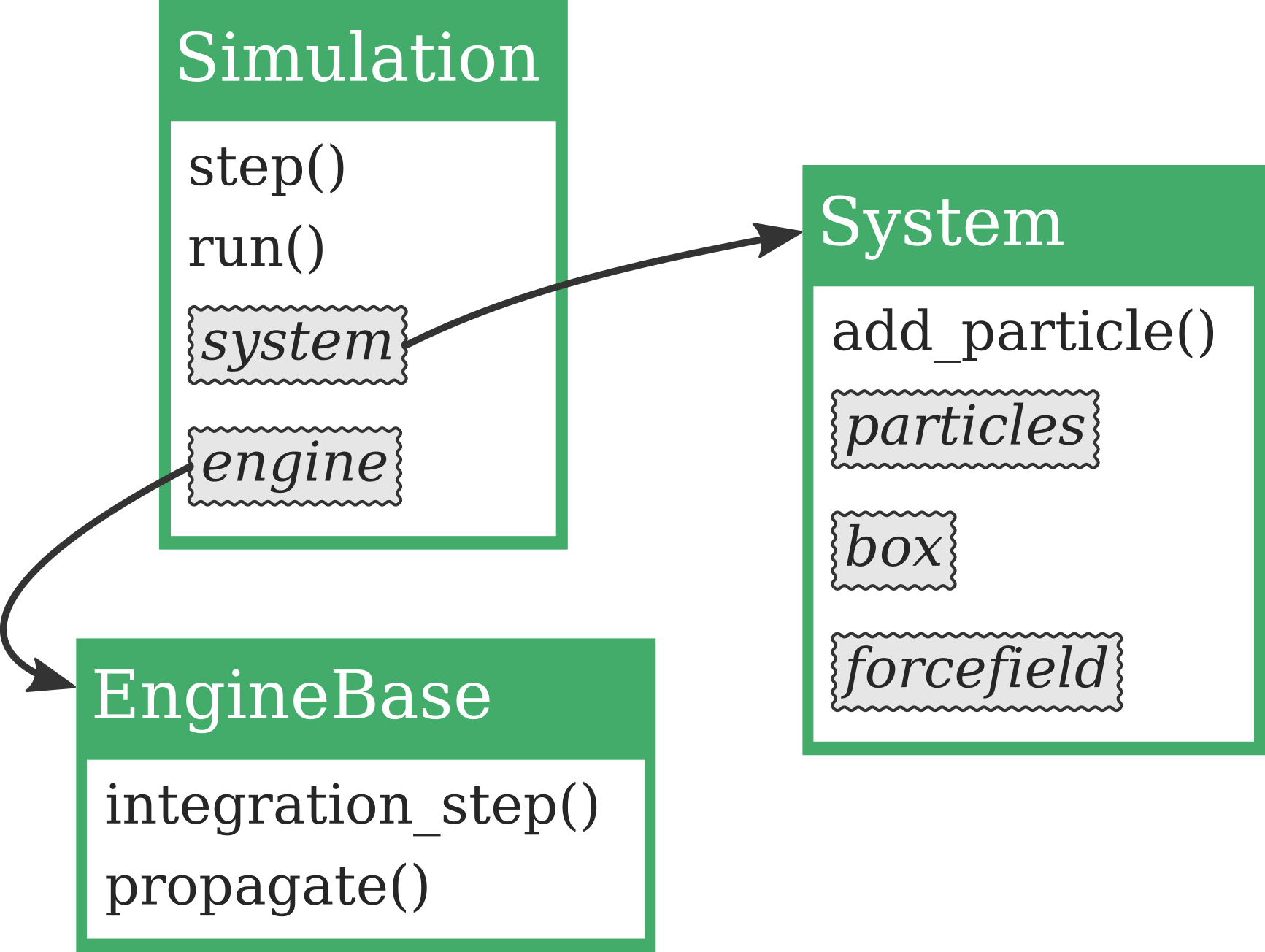Fig. 12 Illustration of the relations between the Simulation, EngineBase and System. A simulation object will typically contain references to a system object and to an engine object. The simulation can then use the engine in order to interact with the system. For instance can the Simulation.step() methods use the EngineBase.integration_step() in order to update positions, velocities and forces in the system.

In this section, we will not give a complete example on how to create a new simulation class or a new engine class. We refer the reader to the examples, in particular:

### The Simulation class¶

The Simulation class defines a simulation we can run. A simulation will typically act on a System and alter its state. We will here just describe the generic base class Simulation and we refer the reader to the extended pyretis API documentation for information about specific simulation classes, for instance, the SimulationRETIS class. The most commonly used methods from the Simulation are:

Example of creation:

from pyretis.simulation import Simulation
new_simulation = Simulation(startcycle=0, endcycle=100)

for step in new_simulation.run():
print(step['cycle']['stepno'])


The code block above will create a generic simulation object and run it. This simulation is not doing anything useful, it will only increment the current simulation step number from the given startcycle to the given endcycle. In order to something more productive, we can attach tasks to the simulation. This can be done as follows:

from pyretis.core import System
from pyretis.simulation import Simulation

def function(sys):
"""Return the set temperature for the system."""
return sys.temperature

simulation = Simulation(steps=10)
system = System(temperature=300)

my_task = {'func': function,
'args': [system],
'first': True,
'result': 'temperature'}

for result in simulation.run():
step = result['cycle']['step']
temp = result['temperature']['set']
print(step, temp)


As you can see, a new task is added by defining it as a dictionary:

my_task = {'func': function,
'args': [system],
'first': True,
'result': 'temperature'}


The following keywords are used:

• func which is a reference to a function to use.
• args which is a list of arguments that should be given to the function.
• first whether the task should be executed on the first simulation step (i.e. step 0).
• result a string which labels the result in the dictionary returned by the methods Simulation.run() or Simulation.step().

Typically, when creating a custom simulation, you will rewrite the methods Simulation.run() and Simulation.step() to fit the custom simulation you are going to perform, rather than adding tasks. However, for interactive work, short examples etc., the Simulation.add_task() can be useful.

### The EngineBase class¶

The EngineBase is a base class defining a generic engine. In PyRETIS engines are either internal or external. External engines, e.g. ExternalMDEngine, interfaces external programs while internal engines, e.g. MDEngine interact directly with a System object. Creating an external engine may be somewhat involved depending on the program you wish to interface. A description on how to create new external engines can be found in the user guide.

## The orderparameter sub-package¶

The pyretis.orderparameter package defines order parameters to use for path sampling simulation. In PyRETIS, such order parameters are assumed to be functions of a System only. Here, we give some examples of how a generic order parameter can be used. For information on how custom order parameters can be created, we refer to the detailed description in the user guide.

### The OrderParameter class¶

The most important piece of the OrderParameter class is the actual calculation of the order parameter. This should be defined in a method like OrderParameter.calculate(). Here, it is assumed that the order parameter takes in an object like System. Since this is described elsewhere we will here just describe the usage of:

First, we create an order parameter. For simplicity we use the pre-defined Position class:

from pyretis.core import System, Particles
from pyretis.orderparameter import Position

position = Position(0, dim='x')


Next, we can calculate the order parameter as follows (the system we set up here is just for testing):

# Define a simple system for testing:
system = System()
system.particles = Particles(dim=3)
system.add_particle([1.0, 2.0, 3.0], mass=1.0, name='Ar', ptype=0)
print('Order =', position.calculate(system))


Several order parameters can be combined by creating a CompositeOrderParameter. Below is an example of how this can be used:

# -*- coding: utf-8 -*-
# Copyright (c) 2015, PyRETIS Development Team.
"""Example of interaction with a Composite order parameter."""
import numpy as np
from pyretis.core import System, Particles
from pyretis.orderparameter import (
OrderParameter,
Position,
CompositeOrderParameter,
)

# Create a new, empty, order parameter:
order_parameter = CompositeOrderParameter()

# Add a position order parameter:

# Add a custom order parameter:
position_y = OrderParameter(description='Position along y-axis')

def collective_y(system):
"""Position along y-axis."""
return [system.particles.pos]

position_y.calculate = collective_y

# Add another order parameter:
position_cos = OrderParameter(description='Cosine of z-coordinate')

def collective_cos(system):
return [np.cos(np.pi * system.particles.pos)]

position_cos.calculate = collective_cos

# Create a system for testing the new order parameter:
system = System()
system.particles = Particles(dim=3)
system.add_particle([1.0, 2.0, 3.0], mass=1.0, name='Ar', ptype=0)
print('Order =', order_parameter.calculate(system))


Important

The first order parameter returned from calculate() is taken as the progress coordinate used in path sampling simulations.

## The analysis sub-package¶

The pyretis.analysis sub-package defines tools which are used in the analysis of simulation output. It defines methods for running predefined analysis tasks, e.g. retis_flux(), but also generic analysis methods, e.g. running_average(). Here, we refer the reader to the pyretis API documentation for more information about these methods.

## The inout sub-package¶

The pyretis.inout sub-package contains methods which PyRETIS make use of in order to interact with you. These are ways to read input from you and to present output to you. This sub-package is relatively large and it is in fact made up by the following sub-packages:

Again, we refer to the pyretis API documentation for more information about these sub-packages.

## The initiation sub-package¶

The pyretis.initiation sub-package contains methods to initialize path ensembles. We refer the reader to the pyretis API documentation for more information about this sub-package.

## The tools sub-package¶

The tools library can be used to generate initial structures for a simulation. In the tools library the function generate_lattice() is defined and it supports the creation of the following lattices where the shorthand keywords (sc, sq etc.) are used to select a specific lattice:

• sc: A simple cubic lattice.
• sq: Square lattice (2D) with one atom in the unit cell.
• sq2: Square lattice with two atoms in the unit cell.
• bcc: Body-centered cubic lattice.
• fcc: Face-centered cubic lattice.
• hcp: Hexagonal close-packed lattice.
• diamond: Diamond structure.

A lattice is generated in the following way:

from pyretis.tools import generate_lattice
xyz, size = generate_lattice('diamond', [1, 1, 1], lcon=3.57)


Where the first parameter selects the lattice type, the second parameter selects give the number of repetitions in each direction and the optional parameter lcon is the lattice constant. The returned values are xyz – the coordinates – and size which is the bounding box of the generated structure. This variable can be used to define a simulation box. It is also possible to specify a number density:

from pyretis.tools import generate_lattice
xyz, size = generate_lattice('diamond', [1, 1, 1], density=1)


If the density is specified, the lattice constant lcon is deduced: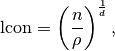where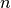is the number of particles in the unit cell,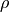the specified number density and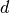the dimensionality. If we wish to save a generated lattice, this can be done as follows

from pyretis.tools import generate_lattice
from pyretis.inout.formats.xyz import write_xyz_file
xyz, size = generate_lattice('diamond', [3, 3, 3], lcon=3.57)
write_xyz_file('diamond.xyz', xyz, names=['C']*len(xyz), header='Diamond')

xyz, size = generate_lattice('hcp', [3, 3, 3], lcon=2.5)
name = ['A', 'B'] * (len(xyz) // 2)
write_xyz_file('hcp.xyz', xyz, names=name, header='HCP test')

xyz, size = generate_lattice('sq2', [3, 3], lcon=1.0)
write_xyz_file('sq2.xyz', xyz, header='sq2 test')


## Some examples of using the PyRETIS library¶

Here, we show some examples of how we can perform some common tasks using the PyRETIS library.

### Reversing a trajectory¶

PyRETIS will not reverse backward trajectories during a simulation if you are using an external engine. For visualization purposes, it is very helpful to reverse these trajectories before viewing them. This can be accomplished with the PyRETIS library as follows:

• For GROMACS .trr trajectories:

from pyretis.inout.formats.gromacs import reverse_trr
reverse_trr('trajB.trr', 'rev-trajB.trr')


which will read the trajectory trajB.trr and store it as rev-trajB.trr.

• For .xyz trajectories:

from pyretis.inout.formats.xyz import reverse_xyz_file
reverse_xyz_file('trajB.xyz', 'rev-trajB.xyz')


which will read the trajectory trajB.xyz and store it as rev-trajB.xyz.

### Recalculating order parameters¶

If you have an existing trajectory and with to calculate order parameters for each step in the trajectory, this can be accomplished by making use of the pyretis.tools.recalculate_order module. For example, if you have a trajectory named traj.trr and an order parameter defined in an input file retis.rst this can be done as follows:

from pyretis.inout.formats.order import OrderFile
from pyretis.inout.settings import parse_settings_file
from pyretis.inout.setup import create_orderparameter
from pyretis.tools.recalculate_order import recalculate_order

settings = parse_settings_file('retis.rst')
order_parameter = create_orderparameter(settings)
options = {'reverse': False, 'maxidx': None, 'minidx': None}
order = recalculate_order(order_parameter, 'traj.trr', options)

with OrderFile('order.txt', 'w') as outfile:
outfile.write('# Order parameters')
for step, data in enumerate(order):
print(step, data)
outfile.output(step, data)


This will create a new file order.txt with the re-calculated order parameters. The keyword reverse can be used to tell PyRETIS that you are looking at a time-reversed trajectory. The keyword minidx gives the frame number from which we start calculating (None is equal to reading from the first frame) and the keyword maxidx gives the last frame number we will read (setting it to None will read until the end of the trajectory file).